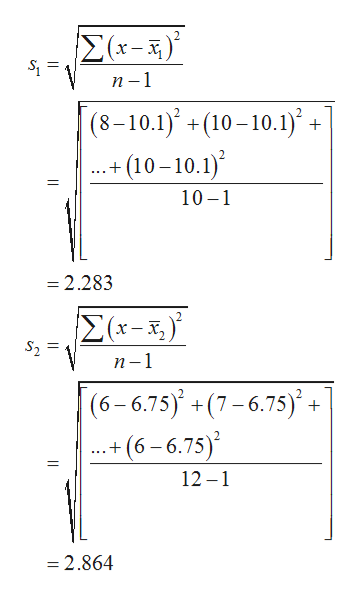# Ten COS Math 21 students were asked how many hours they studied for their final. Here are their responses.8 10 12 7 9 13 8 10 14 10Twelve COS Math 200 students were asked the same questions. Here are their responses. 6 7 5 8 10 68 5 0 11 9 6At the 0.05 level of significance, test the claim that the mean number of hours studied by Math 21 students is greater than the mean number of hours studied by Math 200 students.

Question
10 views

Ten COS Math 21 students were asked how many hours they studied for their final. Here are their responses.

8 10 12 7 9 13 8 10 14 10

Twelve COS Math 200 students were asked the same questions. Here are their responses. 6 7 5 8 10 6
8 5 0 11 9 6

At the 0.05 level of significance, test the claim that the mean number of hours studied by Math 21 students is greater than the mean number of hours studied by Math 200 students.

check_circle

Step 1

Solution:

It is given that the number of samples of Math 21 students is 10 and number of samples of Math 200 students is 12.

Level of significance value is 0.05.

The sample mean of Math 21 students is obtained below:

x̄1=(sum of all observations)/sample size=10.1.

The sample mean of Math 200 students is obtained below:

x̄2=(sum of all observations)/sample size=6.75.

Step 2

The sample standard deviations of Math 21 students and Math 200 students are obtained below:

The s1 and s2 be the sample standard deviations of Math 21 students and Math 200 students.help_outlineImage TranscriptioncloseΣκ-3). S = п-1 (8–10.1)° +(10 –10.1)° + .+ (10 – 10.1) .. 10 -1 = 2.283 Σ(-) х— х, S2 п-1 |(6-6.75) +(7-6.75)' + .+ (6 – 6.75) 12 –1 = 2.864 || || fullscreen
Step 3

The null and alternative hypotheses are given below:

Let µ1 be the mean of Math 21 students and µ2 be the mean of Math 200 students

Null hypothesis:

H0:µ1= µ2

Alternative hypothesis:

H1:µ1>µ2

...

### Want to see the full answer?

See Solution

#### Want to see this answer and more?

Solutions are written by subject experts who are available 24/7. Questions are typically answered within 1 hour.*

See Solution
*Response times may vary by subject and question.
Tagged in

### Statistics